## 为什么你该摒弃 Java ，全面转向 Kotlin 语言？

Kotlin 是 JetBrains 开发的，这是一家开发了一整套 IDEs 的公司，诸如 IntelliJ 和 ReSharper, 还有正在闪耀光芒的 Kotlin。这是一个务实而且简洁的编程语言，真正让人感觉身心愉悦的编程语言，而且效率非常高。

### 0# Java 互操作性

Kotlin 是 100% 与 Java 具备互操作性的。你可以使用 Kotlin 继续你老的 Java 项目开发。所有你熟悉的 Java 框架仍然可用，任何框架，不管是 Kotlin 写的，还是你固执 Java 小伙伴写的都可以。

### 1# 熟悉的语法

Kotlin 不是诞生于学术界的怪异语言。其语法是 OOP 领域的任何开发者都熟悉的，可以或多或少的有一些了解。当然和 Java 还是有些不同的，例如重构后的构造函数以及使用 val 的变量声明等等。下面是一个基本的 Kotlin 示例代码：

```class Foo {

val b: String = "b"     // val means unmodifiable
var i: Int = 0          // var means modifiable

fun hello() {
val str = "Hello"
print("\$str World")
}

fun sum(x: Int, y: Int): Int {
return x + y
}

fun maxOf(a: Float, b: Float) = if (a > b) a else b

}```

### 2# 字符串插值

`val x = 4val y = 7print("sum of \$x and \$y is \${x + y}")  // sum of 4 and 7 is 11`

### 3# 类型推断

Kotlin 会自动的对变量的类型进行推断：

`val a = "abc"                         // type inferred to Stringval b = 4                             // type inferred to Intval c: Double = 0.7                   // type declared explicitlyval d: List<String> = ArrayList()     // type declared explicitly`

### 4# 智能类型转换

Kotlin 编译器会跟踪你的逻辑代码并在需要的时候进行自动的类型转换，这意味着我们不需要在显示转换时做更多的 instanceof 检查：

```if (obj is String) {    print(obj.toUpperCase())     // obj is now known to be a String
}```

### 5# 更直观的相等性比较

```val john1 = Person("John")
val john2 = Person("John")```
```john1 == john2    // true  (structural equality)
john1 === john2   // false (referential equality)```

### 6# 默认参数值

```fun build(title: String, width: Int = 800, height: Int = 600) {
Frame(title, width, height)
}```

### 7# 命名参数

```build("PacMan", 400, 300)                           // equivalent
build(title = "PacMan", width = 400, height = 300)  // equivalent
build(width = 400, height = 300, title = "PacMan")  // equivalent```

### 8# When 表达式

switch 替换成 when ，代码更具可读性：

```when (x) {
1 -> print("x is 1")
2 -> print("x is 2")
3, 4 -> print("x is 3 or 4")    in 5..10 -> print("x is 5, 6, 7, 8, 9, or 10")    else -> print("x is out of range")
}```

```val res: Boolean = when {
obj == null -> false
obj is String -> true    else -> throw IllegalStateException()
}```

### 9# Properties

```class Frame {    var width: Int = 800    var height: Int = 600    val pixels: Int        get() = width * height
}```

### 10# Data 类

`data class Person(val name: String,                  var email: String,                  var age: Int)val john = Person("John", "john@gmail.com", 112)`

### 11# 操作符重载

```data class Vec(val x: Float, val y: Float) {    operator fun plus(v: Vec) = Vec(x + v.x, y + v.y)
}val v = Vec(2f, 3f) + Vec(4f, 1f)```

### 12# 解构声明

```for ((key, value) in map) {    print("Key: \$key")    print("Value: \$value")
}```

### 13# Ranges

```for (i in 1..100) { ... }
for (i in 0 until 100) { ... }for (i in 2..10 step 2) { ... }
for (i in 10 downTo 1) { ... }
if (x in 1..10) { ... }```

### 14# 扩展函数

```fun String.replaceSpaces(): String {    return this.replace(' ', '_')
}val formatted = str.replaceSpaces()```

```str.removeSuffix(".txt")
str.capitalize()
str.substringAfterLast("/")
str.replaceAfter(":", "classified")```

### 15# Null 安全

Java 是我们应该称之为是一个几乎静态类型的编程语言。Java 的 String 变量类型无法保证它不会等于 null。尽管我们已经习惯了这样的情况，但它否定了静态类型检查的安全性，导致 Java 程序员总是活在各种空指针异常的恐惧中。

Kotlin 通过区分非空类型和允许为空类型来解决这个问题。类型默认是不允许为空的，可以通过在后面加一个 ? 问号来表示允许为空，例如：

```var a: String = "abc"
a = null                // 编译错误var b: String? = "xyz"
b = null                // 正确```

Kotlin 强制你必须在访问一个可为空的类型变量时候必须确保不会发生空指针：

`val x = b.length        // 编译错误: b 允许为空`

`if (b == null) returnval x = b.length        // 正确`

`val x = b?.length       // type of x is nullable Int`

`val name = ship?.captain?.name ?: "unknown"`

`val x = b?.length ?: throw NullPointerException()  // same as belowval x = b!!.length                                 // same as above`

### 16# 更好的 Lambdas

`val sum = { x: Int, y: Int -> x + y }   // type: (Int, Int) -> Intval res = sum(4,7)                      // res == 11`

1. 如果 lambda 是方法的最后一个参数或者是唯一参数的话，那么方法的括号可以移动或者省略.

2. 如果我们选择不去声明单参数的 lambda 表达式的参数，那么 Kotlin 会隐式的将之命名为 `it`.

```numbers.filter({ x -> x.isPrime() })
numbers.filter { x -> x.isPrime() }
numbers.filter { it.isPrime() }```

```persons
.filter { it.age >= 18 }
.sortedBy { it.name }
.map { it.email }
.forEach { print(it) }```

Kotlin 的 lambda 系统和扩展函数结合，可以非常棒的用来开发各种 DSL。例如 Anko 这个 DSL 的例子可以增强 Android 的开发：

```verticalLayout {
editText {
hint = “Name”
textSize = 24f
}
editText {
textSize = 24f
}
textSize = 26f
}
}```

### 17# IDE 支持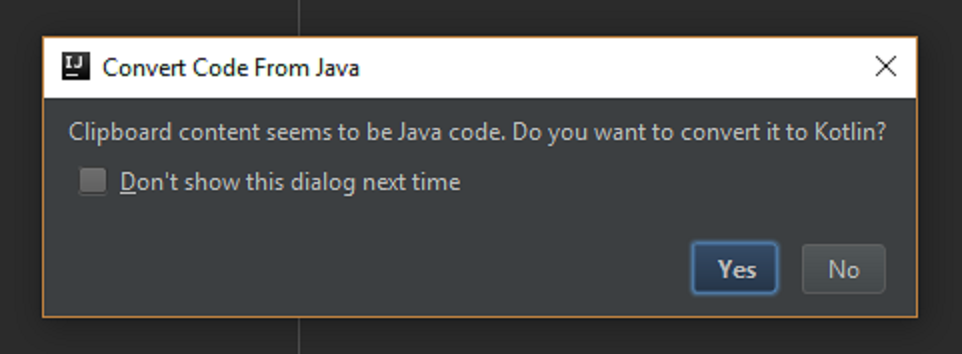IntelliJ 会提醒你粘贴了 Java 的代码到 Kotlin 文件里。

### 精彩评论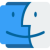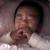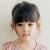### 最新评论（129）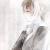#### 引用来自“双城记”的评论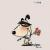#### 引用来自“双城记”的评论

c